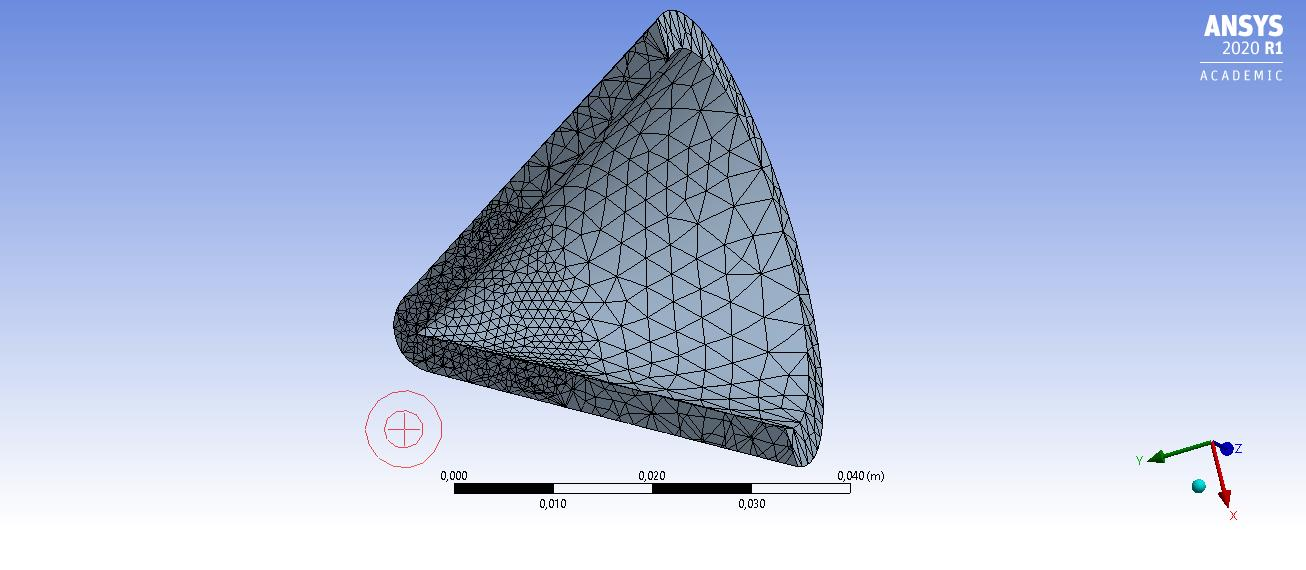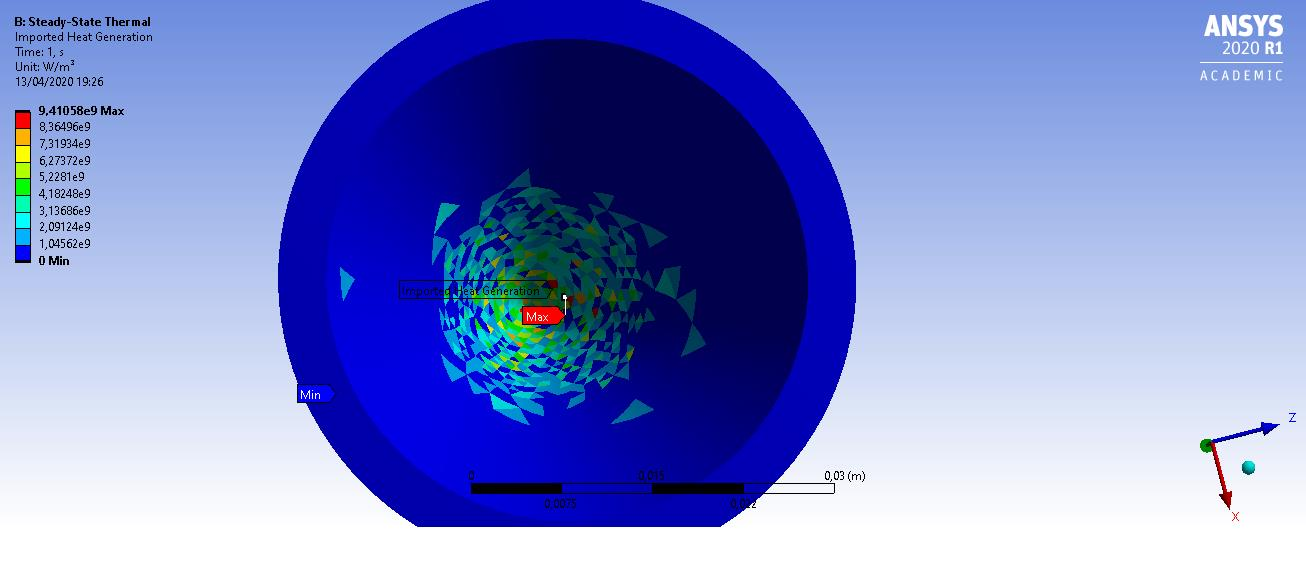# Import heat generation into a different mesh

Hello all,

I am trying to calculate how a Faraday Cup heats when the particle beam impacts on it. First I calculate the energy deposit using Geant4. Then, I import the energy deposition of the beam (in W/mm^3) in a 3D rectangular cubic mesh, in which the energy deposition in each cube is defined in the center of it.  I can freely change the sizes of these cubes, but not the shape. The Faraday Cup, however, has a conical geometry (image attached) and the mesh to do the calculation is adjusted to the geometry of the body.  Thus, the shapes of the imported energy deposit mesh and the mesh to do the calculations are very different.

Now comes the problem, when I import the heat generation, ANSYS tries to adjust it to its mesh. When I check the total imported load (integral of the heat genetarion in all the volume) I see that ANSYS has imported 450W instead of the 700W that my source file has, so there is a loss of heat in the adjustement between both different meshes. I have tried different types of manual mappings, but none of them has improved the situation substantially. What I would like ANSYS to do is that once the mesh of the conical shape is defined, to check how many points of the original cubic mesh fall inside each element of the ANSYS mesh and to add them, correcting for the volume of the new mesh element. I would greatly appreciate if someone could let me know how to solve this problem, or at least to help me understand how ANSYS transfers the information from the original file to its own mesh. I attach an image of the geometry of the Faraday Cup with the mesh defined in ANSYS and the imported heat generation.

Thank you,